Search
•Nagesh Singh Chauhan

# Introduction to Restricted Boltzmann Machines(RBMs)

The article contains intuition behind Restricted Boltzmann Machines — A powerful Tool for Recommender Systems.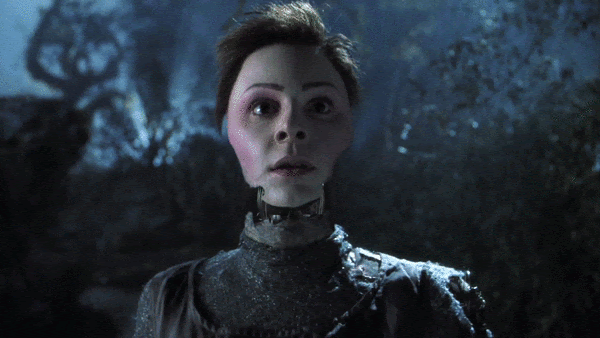## Introduction

Invented by Geoffrey Hinton(Sometimes referred to as the Godfather of Deep Learning), a Restricted Boltzmann machine is an algorithm useful for dimensionality reduction, classification, regression, collaborative filtering, feature learning, and topic modeling.

Before moving forward let us first understand what is Boltzmann Machines?

## What are Boltzmann Machines?

A Boltzmann machine is a stochastic(non-deterministic) or generative deep learning model which has only visible(input) and hidden nodes.

The image below presents ten nodes in it and all of them are inter-connected and are also often referred to as States. Brown ones represent Hidden nodes (h)and blue ones represent Visible nodes (v). If you already understand Artificial, Convolutional, and Recurrent Neural networks, you’ll notice they never had their Input nodes connected, whereas Boltzmann Machines have their inputs connected & that is what makes them fundamentally unconventional. All these nodes exchange information among themselves and self-generate subsequent data hence termed as Generative deep model.Boltzmann machine hidden and visible nodes

There is no output node in this model hence like our other classifiers, we cannot make this model learn 1 or 0 from the Target variable of the training dataset after applying gradient descent or stochastic gradient descent (SGD), etc. Exactly similar cases with our regressor models as well, where it cannot learn the pattern from Target variables. These attributes make the model non-deterministic. Thinking of how does this model then learns and predicts, is that intriguing enough?

Here, Visible nodes are what we measure and Hidden nodes are what we don’t measure. When we input data, these nodes learn all the parameters, their patterns, and correlation between those on their own and forms an efficient system, hence Boltzmann Machine is termed as an Unsupervised Deep Learning model. This model then gets ready to monitor and study abnormal behavior depending on what it has learned.

Hinton once referred to the illustration of a Nuclear Power plant as an example for understanding Boltzmann Machines. This is a complex topic so we shall proceed slowly to understand the intuition behind each concept, with a minimum amount of mathematics and physics involved.So in the simplest introductory terms, Boltzmann Machines are primarily divided into two categories: Energy-based Models (EBMs) and Restricted Boltzmann Machines (RBMs). When these RBMs are stacked on top of each other, they are known as Deep Belief Networks (DBNs).

## What are Restricted Boltzmann Machines?

A Restricted Boltzmann Machine (RBM) is a generative, stochastic, and 2-layer artificial neural network that can learn a probability distribution over its set of inputs.

Stochastic means “randomly determined”, and in RBMs, the coefficients that modify inputs are randomly initialized.

The first layer of the RBM is called the visible, or input layer, and the second is the hidden layer. Each circle represents a neuron-like unit called a node. Each node in the input layer is connected to every node of the hidden layer.The restriction in a Restricted Boltzmann Machine is that there is no intra-layer communication(nodes of the same layer are not connected). This restriction allows for more efficient training algorithms than what is available for the general class of Boltzmann machines, in particular, the gradient-based contrastive divergence algorithm. Each node is a locus of computation that processes input and begins by making stochastic decisions about whether to transmit that input or not.

RBM received a lot of attention after being proposed as building blocks of multi-layer learning architectures called Deep Belief Networks(DBNs). When these RBMs are stacked on top of each other, they are known as DBNs.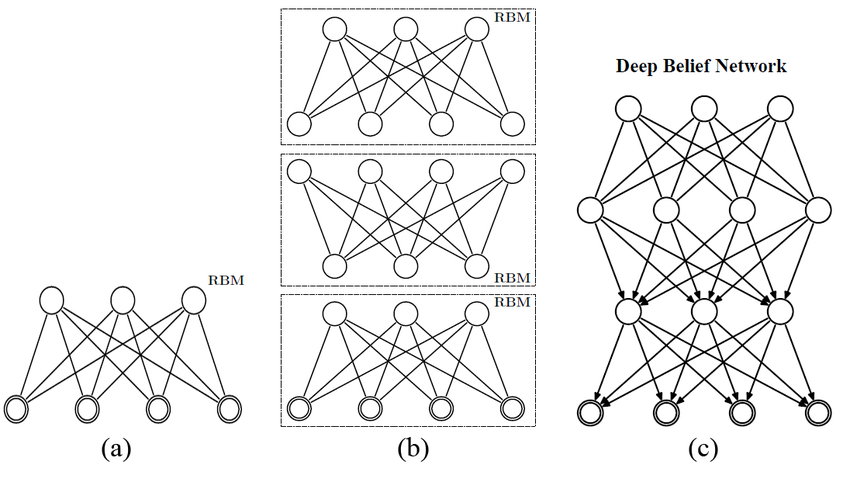## Difference between Autoencoders & RBMs

Autoencoder is a simple 3-layer neural network where output units are directly connected back to input units. Typically, the number of hidden units is much less than the number of visible ones. The task of training is to minimize an error or reconstruction, i.e. find the most efficient compact representation for input data.Layers in Autoencoders

RBM shares a similar idea, but it uses stochastic units with particular distribution instead of deterministic distribution. The task of training is to find out how these two sets of variables are connected.

## Working of Restricted Boltzmann Machine

One aspect that distinguishes RBM from other Neural networks is that it has two biases.

• The hidden bias helps the RBM produce the activations on the forward pass, while

• The visible layer’s biases help the RBM learn the reconstructions on the backward pass.

The reconstructed input is always different from the actual input as there are no connections among visible nodes and therefore, no way of transferring information among themselves.Forward pass

The above image shows the first step in training an RBM with multiple inputs. The inputs are multiplied by the weights and then added to the bias. The result is then passed through a sigmoid activation function and the output determines if the hidden state gets activated or not. Weights will be a matrix with the number of input nodes as the number of rows and the number of hidden nodes as the number of columns. The first hidden node will receive the vector multiplication of the inputs multiplied by the first column of weights before the corresponding bias term is added to it.

The sigmoid function is given by:So the equation that we get in this step would be,where h(1) and v(0) are the corresponding vectors (column matrices) for the hidden and the visible layers with the superscript as the iteration (v(0) means the input that we provide to the network) and a is the hidden layer bias vector.Backward pass

Now this image shows the reverse phase or the reconstruction phase. It is similar to the first pass but in the opposite direction. The equation comes out to be:Where v(1) and h(1) are the corresponding vectors (column matrices) for the visible and the hidden layers with the superscript as the iteration and b is the visible layer bias vector.

## Training a Restricted Boltzmann Machine

The training of the Restricted Boltzmann Machine differs from the training of regular neural networks via stochastic gradient descent(SGD).

The difference v(0)-v(1) can be considered as the reconstruction error that we need to reduce in subsequent steps of the training process. So the weights are adjusted in each iteration to minimize this error and this is what the learning process essentially is.

In the forward pass, we are calculating the probability of output h(1) given the input v(0) and the weights W denoted by:and in the backward pass, while reconstructing the input, we are calculating the probability of output v(1) given the input h(1) and the weights Wdenoted by:The weights used in both the forward and the backward pass are the same. Together, these two conditional probabilities lead us to the joint distribution of inputs and the activations:Reconstruction is different from regression or classification in that it estimates the probability distribution of the original input instead of associating a continuous/discrete value to an input example. This means it is trying to guess multiple values at the same time. This is known as generative learning as opposed to discriminative learning that happens in a classification problem (mapping input to labels).

## Contrastive Divergence (CD-k)

Boltzmann Machines (and RBMs) are Energy-based models and a joint configuration, (v,h) of the visible and hidden units has energy given by:where vi, hj, are the binary states of the visible unit i and hidden unit j, ai, bj are their biases and wij is the weight between them.

The probability that the network assigns to a visible vector vis given by summing over all possible hidden vectors:Z here is the partition function and is given by summing over all possible pairs of visible and hidden vectors:This gives us:The log-likelihood gradient or the derivative of the log probability of a training vector concerning weight is surprisingly simple:where the angle brackets are used to denote expectations under the distribution specified by the subscript that follows. This leads to a very simple learning rule for performing stochastic steepest ascent in the log probability of the training data: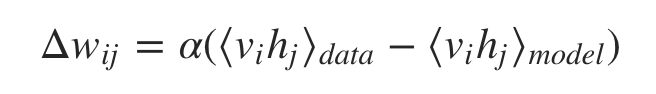where alpha is a learning rate.

For more information on what the above equations mean or how they are derived, refer to the Guide on training RBM by Geoffrey Hinton. The important thing to note here is that because there are no direct connections between hidden units in an RBM, it is very easy to get an unbiased sample of ⟨vi hj⟩ data. Getting an unbiased sample of ⟨vi hj⟩ model, however, is much more difficult. This is because it would require us to run a Markov chain until the stationary distribution is reached (which means the energy of the distribution is minimized — equilibrium!) to approximate the second term. So instead of doing that, we perform Gibbs Sampling from the distribution. It is a Markov chain Monte Carlo (MCMC) algorithm for obtaining a sequence of observations which are approximated from a specified multivariate probability distribution when direct sampling is difficult (like in our case). The Gibbs chain is initialized with a training example v(0) of the training set and yields the sample v(k) after k steps. Each step t consists of sampling h(t) from p(h | v(t)) and sampling v(t+1) from p(v | h(t)) subsequently (the value k = 1 surprisingly works quite well). The learning rule now becomes: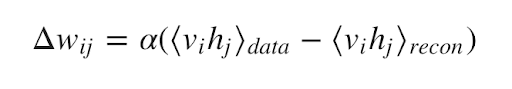The learning works well even though it is only crudely approximating the gradient of the log probability of the training data. The learning rule is much more closely approximating the gradient of another objective function called the Contrastive Divergence which is the difference between two Kullback-Liebler divergences.

When we apply this, we get: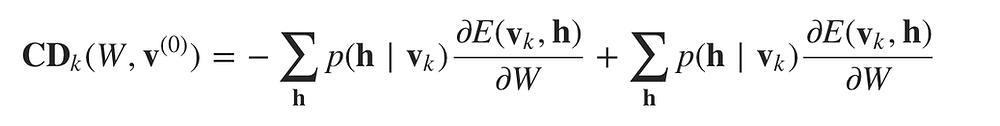Where the second term is obtained after each k steps of Gibbs Sampling.

Now let us understand RBM with the help of an example.

## A practical example of RBM: Collaborative Filtering## Recognizing Latent factors in the Data

Let us assume that some people were asked to rate a set of movies on a scale of 1–5 and each movie could be explained in terms of a set of latent factors(in this case genre) such as action, fantasy, horror, drama, etc. RBMs are used to analyze and find out these underlying latent factors.The analysis of hidden factors is performed in a binary way, i.e, the user only tells if they liked (rating 1) a specific movie or not (rating 0) and it represents the inputs for the input/visible layer. Given the inputs, the RMB then tries to discover latent factors in the data that can explain the movie choices and each hidden neuron represents one of the latent factors.

Let us consider the following example where a user likes Lord of the Rings and Harry Potter but does not like The Matrix, Fight Club, and Titanic. The Hobbit has not been seen yet so it gets a -1 rating. Given these inputs, the RBM may identify three hidden factors Drama, Fantasy, and Science Fiction which correspond to the movie genres.

## Latent Factors for Prediction

After the training RBM, our goal is to predict a binary rating for the movies that had not been seen yet. Given the training data of a specific user, the network can identify the latent factors based on the user’s preference and sample from Bernoulli distribution can be used to find out which of the visible neurons now become active.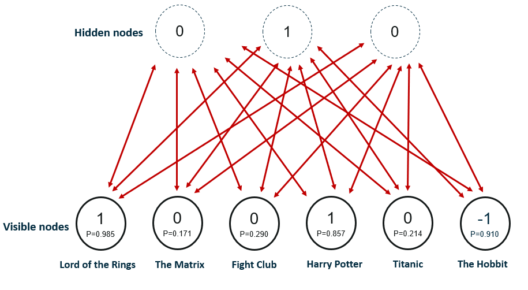The image shows the new ratings after using the hidden neuron values for the inference. The network identified Fantasy as the preferred movie genre and rated The Hobbit as a movie the user would like.

The process from training to the prediction phase goes as follows:

• Train the network on the data of all users

• During inference-time, take the training data of a specific user

• Use this data to obtain the activations of hidden neurons

• Use the hidden neuron values to get the activations of input neurons

• The new values of input neurons show the rating the user would give yet unseen movies.

## Conclusion

You can interpret RBMs’ output numbers as percentages. Every time the number in the reconstruction is not zero, that’s a good indication the RBM learned the input.

It should be noted that RBMs do not produce the most stable, consistent results of all shallow, feedforward networks. In many situations, a dense-layer autoencoder works better. Indeed, the industry is moving toward tools such as variational autoencoders and Generative Adversarial Networks(GAN).

Well, that’s all for this article hope you guys have enjoyed reading it and I’ll be glad if the article is of any help. Feel free to share your thoughts/feedback in the comment section.

## References:

https://towardsdatascience.com/restricted-boltzmann-machines-simplified-eab1e5878976

https://en.wikipedia.org/wiki/Restricted_Boltzmann_machine

https://medium.com/@neuralnets/boltzmann-machines-transformation-of-unsupervised-deep-learning-part-1-42659a74f530

https://www.edureka.co/blog/restricted-boltzmann-machine-tutorial/

https://wiki.pathmind.com/restricted-boltzmann-machine